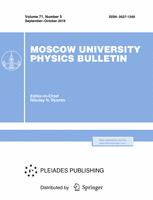Faculty of Physics
M.V.Lomonosov Moscow State University
Regular Article

# Method of optical registration of acoustic field in liquids

## S.A. Petrosyan$^1$, S.A. Tsysar$^2$ , V.D. Svet$^3$, D.A. Dementev$^2$, A.V. Churenkov $^2$

### Memoirs of the Faculty of Physics 2015. N 4.

• Article
Annotation

The main aim of the work is to create a numerical model of acoustic wave propagation in rigid rod. Vibrations of the end of the rod will be recorded by an optical method. Developed model can help to find the optimal parameters to create a real physical system for the efficient optical registration of the acoustic signal.

PACS:
43.58.+z Acoustical measurements and instrumentation
Authors
S.A. Petrosyan$^1$, S.A. Tsysar$^2$ , V.D. Svet$^3$, D.A. Dementev$^2$, A.V. Churenkov $^2$
$^1$ Department of Photonics and Microwave Physics, Faculty of Physics, Lomonosov Moscow State University. Moscow 119991, Russia.
$^2$ N.N. Andreyev Acoustic Institute, Moscow 117036, Russia.
$^3$ Moscow Institute of Physics and Technology (State University), Moscow 117303, Russia.### Moscow University Physics Bulletin### Science News of the Faculty of Physics, Lomonosov Moscow State University

This new information publication, which is intended to convey to the staff, students and graduate students, faculty colleagues and partners of the main achievements of scientists and scientific information on the events in the life of university physicists.